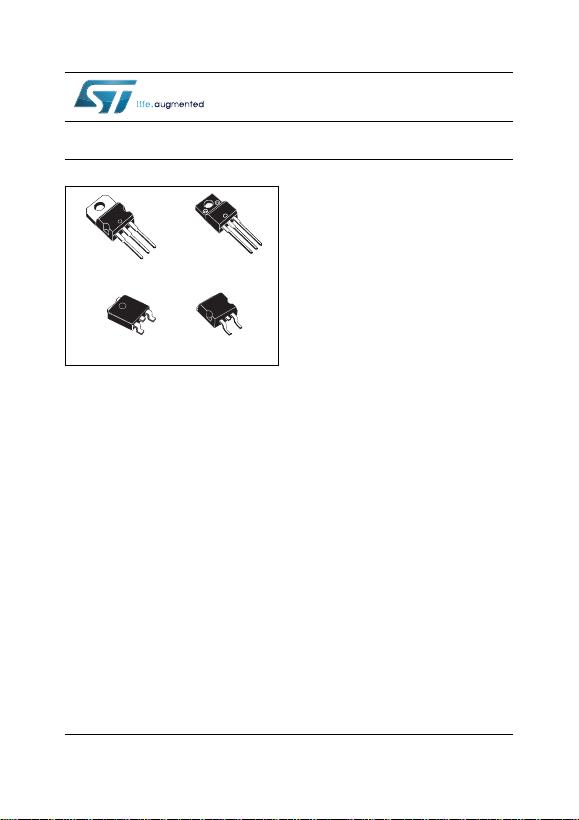This is information on a product in full production.
February 2016 DocID2143 Rev 33 1/59
L78
Positive voltage regulator ICs
Datasheet
-
production data
Features
Output current up to 1.5 A
Output voltages of 5; 6; 8; 8.5; 9; 12; 15; 18;
24 V
Short circuit protection
Output transition SOA protection
2 % output voltage tolerance (A version)
Guaranteed in extended temperature range
(A version)
Description
The L78 series of three-terminal positive
regulators is available in TO-220, TO-220FP,
D²PAK and DPAK packages and several fixed
output voltages, making it useful in a wide range
of applications.
These regulators can provide local on-card
regulation, eliminating the distribution problems
associated with single point regulation. Each type
embeds internal current limiting, thermal shut-
down and safe area protection, making it
essentially indestructible. If adequate heat sinking
is provided, they can deliver over 1 A output
current. Although designed primarily as fixed
voltage regulators, these devices can be used
with external components to obtain adjustable
voltage and currents.
TO-220FPTO-220
D²PAK
DPAK
www.st.com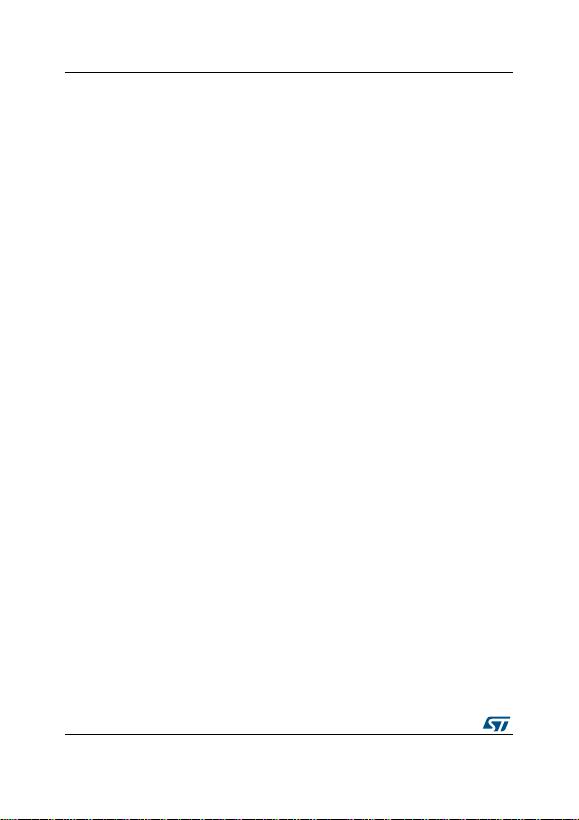Contents L78
2/59 DocI D2143 Rev 33
Contents
1 Diagram . . . . . . . . . . . . . . . . . . . . . . . . . . . . . . . . . . . . . . . . . . . . . . . . . . . 3
2 Pin configuration . . . . . . . . . . . . . . . . . . . . . . . . . . . . . . . . . . . . . . . . . . . 4
3 Maximum ratings . . . . . . . . . . . . . . . . . . . . . . . . . . . . . . . . . . . . . . . . . . . . 5
4 Test circuits . . . . . . . . . . . . . . . . . . . . . . . . . . . . . . . . . . . . . . . . . . . . . . . . 6
5 Electrical characteristics . . . . . . . . . . . . . . . . . . . . . . . . . . . . . . . . . . . . . 7
6 Application information . . . . . . . . . . . . . . . . . . . . . . . . . . . . . . . . . . . . . 23
6.1 Design consideration . . . . . . . . . . . . . . . . . . . . . . . . . . . . . . . . . . . . . . . . 23
7 Typical performance . . . . . . . . . . . . . . . . . . . . . . . . . . . . . . . . . . . . . . . . 33
8 Package information . . . . . . . . . . . . . . . . . . . . . . . . . . . . . . . . . . . . . . . . 35
8.1 T O-220 (dual gauge) package info rmation . . . . . . . . . . . . . . . . . . . . . . . . 36
8.2 TO-220 (single gauge) package information . . . . . . . . . . . . . . . . . . . . . . 38
8.3 T O-220FP package information . . . . . . . . . . . . . . . . . . . . . . . . . . . . . . . . 40
8.4 TO-220 packing information . . . . . . . . . . . . . . . . . . . . . . . . . . . . . . . . . . . 42
8.5 DPAK package information . . . . . . . . . . . . . . . . . . . . . . . . . . . . . . . . . . . 43
8.6 DPAK packing information . . . . . . . . . . . . . . . . . . . . . . . . . . . . . . . . . . . . 46
8.7 D²PAK (SMD 2L STD-ST) type A package information . . . . . . . . . . . . . . 48
8.8 D²PAK (SMD 2L Wooseok-subcon.) package information . . . . . . . . . . . . 50
8.9 D²PAK packing information . . . . . . . . . . . . . . . . . . . . . . . . . . . . . . . . . . . 53
9 Ordering information . . . . . . . . . . . . . . . . . . . . . . . . . . . . . . . . . . . . . . . 55
10 Revision history . . . . . . . . . . . . . . . . . . . . . . . . . . . . . . . . . . . . . . . . . . . 57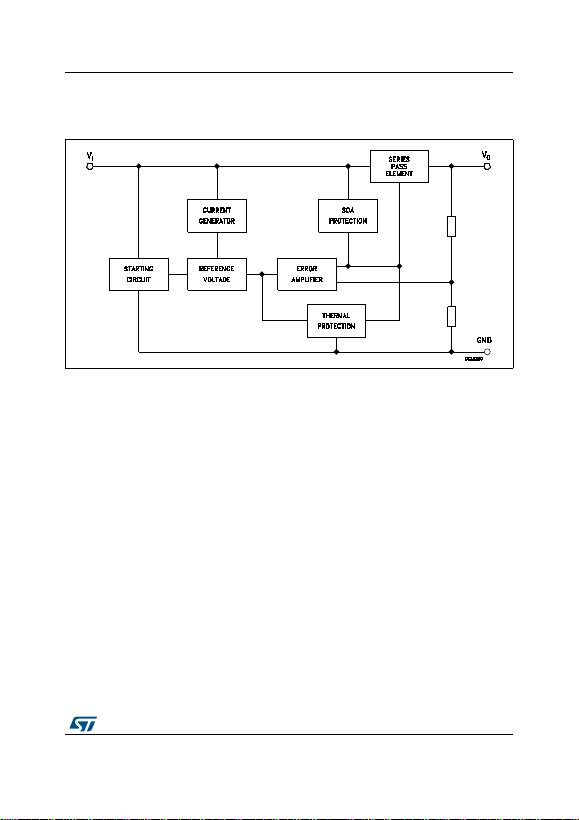DocID2143 Rev 33 3/59
L78 Diagram
59
1 Diagram
Figure 1. Block diagram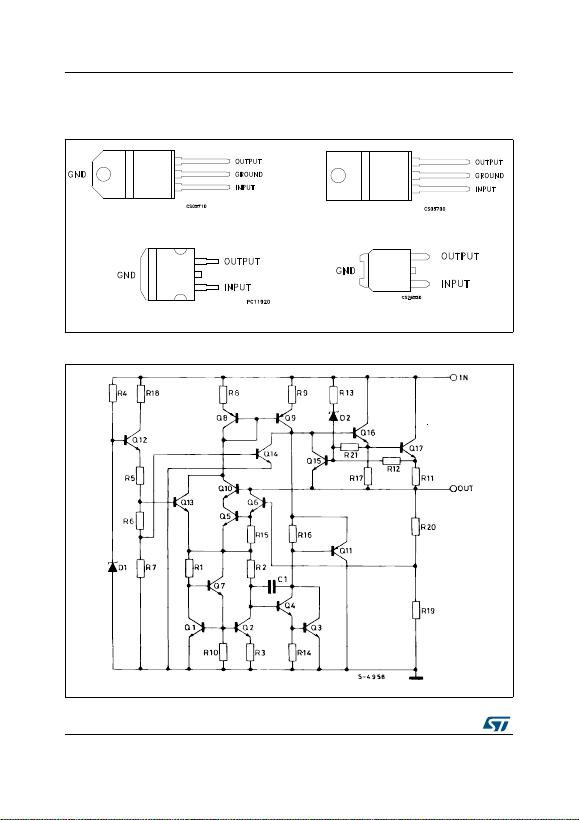Pin configuration L78
4/59 DocI D2143 Rev 33
2 Pin configuration
Figure 2. Pin connections (top view)
72)3
72
'ð3\$. '3\$.
Figure 3. Schematic diag ram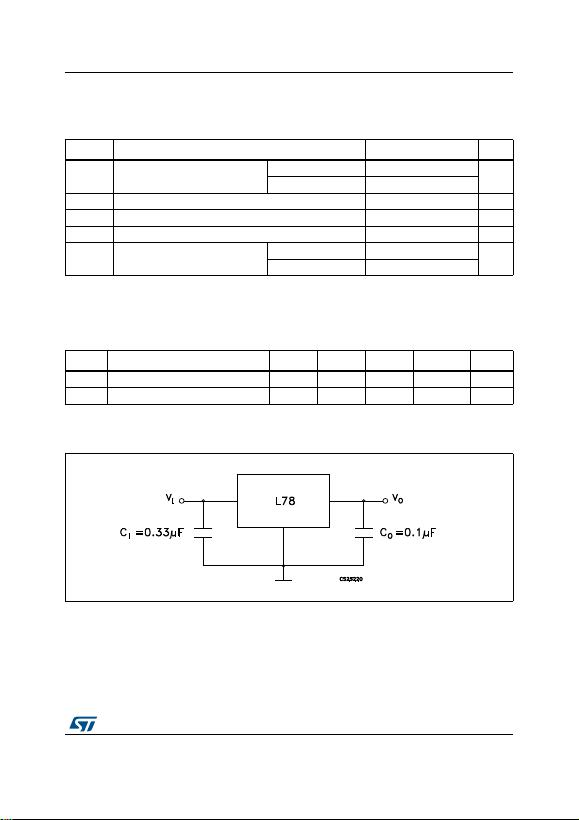DocID2143 Rev 33 5/59
L78 Maximum ratings
59
3 Maximum ratings
Note: Absolute maximum ratings are those values beyond which damage to the device may occur.
Functional operation under these condition is not implied.
Table 1. Absolute maximum ratings
Symbol Parameter Value Unit
V
I
DC input voltage for V
O
= 5 to 18 V 35 V
for V
O
= 20, 24 V 40
I
O
Output current Internally limited
P
D
Power dissipation Internally limited
T
STG
Storage temperature range -65 to 150 °C
T
OP
Operating junction temperature range for L78xxC, L78xxAC 0 to 125 °C
for L78xxAB -40 to 125
Table 2. Thermal data
Symbol Parameter D²PAK DPAK TO-220 TO-220FP Unit
R
thJC
Thermal resistance junction-case 3 8 5 5 °C/W
R
thJA
Thermal resistance junction-ambient 62.5 100 50 60 °C/W
Figure 4. Application circuits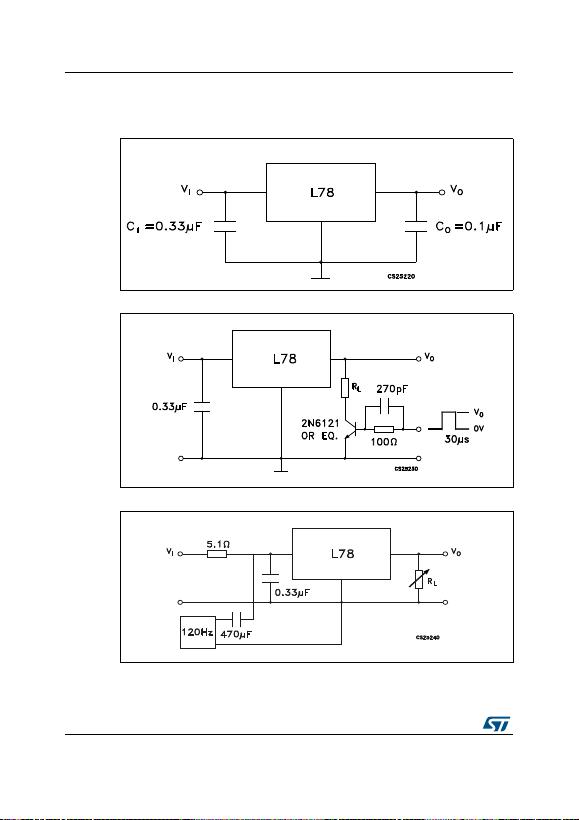Test circuits L78
6/59 DocI D2143 Rev 33
4 Test circuits
Figure 5. DC parameter
Figure 7. Ripple rejection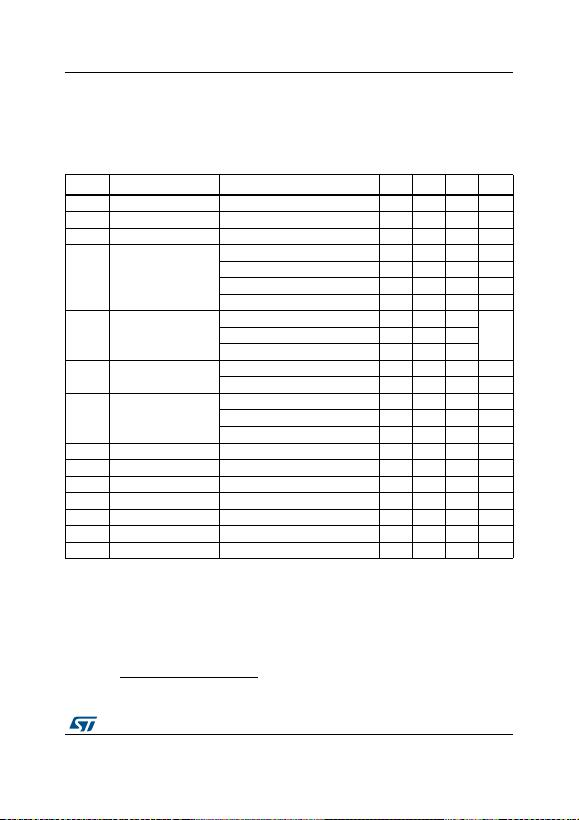DocID2143 Rev 33 7/59
L78 Electrical ch ara ct er istics
59
5 Electrical characteristics
V
I
= 10 V, I
O
= 1 A, T
J
= 0 to 125 °C (L7805AC), T
J
= -40 to 125 °C (L7805AB), unl ess
otherwise specified
(a)
.
a. Minimum load current for regulation is 5 mA.
Table 3. Electrical characteristics of L7805A
Symbol Parameter Test conditions Min. Typ. Max. Unit
V
O
Output voltage T
J
= 25°C 4.9 5 5.1 V
V
O
Output voltage I
O
= 5 mA to 1 A, V
I
= 7.5 to 18 V 4.8 5 5.2 V
V
O
Output voltage I
O
= 1 A, V
I
= 18 to 20 V, T
J
= 25°C 4 .8 5 5.2 V
ΔV
O (1)
Line regulation
V
I
= 7.5 to 25 V, I
O
= 500 mA, T
J
= 25°C 7 50 mV
V
I
= 8 to 12 V 10 50 mV
V
I
= 8 to 12 V, T
J
= 25°C 2 25 mV
V
I
= 7.3 to 20 V, T
J
= 25°C 7 50 mV
ΔV
O (1)
I
O
= 5 mA t o 1 A 25 100
mVI
O
= 5 mA to 1.5 A, T
J
= 25°C 30 100
I
O
= 250 to 750 mA 8 50
I
q
Quiescent current T
J
= 25°C 4.3 6 mA
6mA
ΔI
q
Quiescent current change
V
I
= 8 to 23 V, I
O
= 500 mA 0.8 mA
V
I
= 7.5 to 20 V, T
J
= 25°C 0.8 mA
I
O
= 5 mA to 1 A 0.5 mA
SVR Supply voltage rejection V
I
= 8 to 18 V, f = 120 Hz, I
O
= 500 mA 68 dB
V
d
Dropout voltage I
O
= 1 A, T
J
= 25°C 2 V
eN Output noise voltage T
A
= 25°C, B =10 Hz to 100 kHz 10 µV/V
O
R
O
Output resistance f = 1 kHz 17 mΩ
I
sc
Short circuit current V
I
= 35 V, T
A
= 25°C 0.2 A
I
scp
Short circuit peak current T
J
= 25°C 2.2 A
ΔV
O
/ΔT Output voltage drift -1.1 mV/°C
1. Load and line regulation are specified at const ant junction temperature. Changes in V
O
due to heating effects must be
taken into account separately. Pulse testing with low duty cycle is used.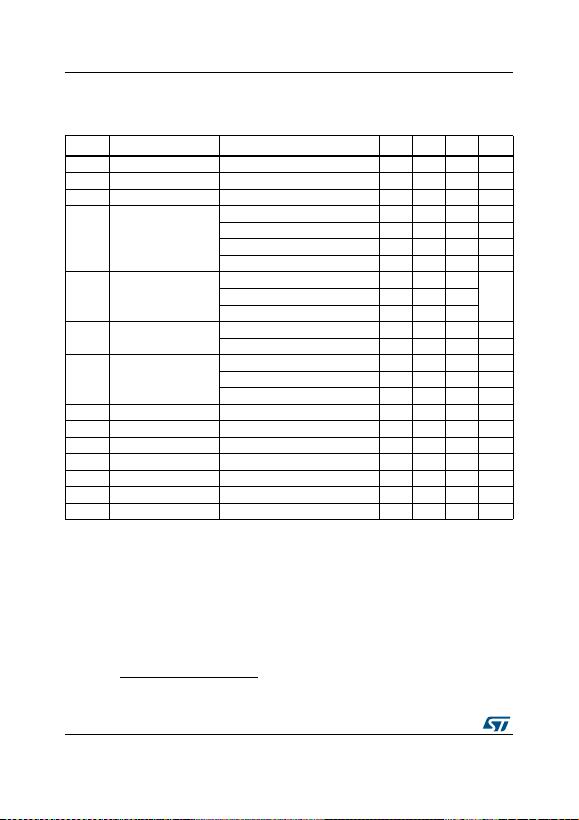Ele ctrical characteristics L78
8/59 DocI D2143 Rev 33
V
I
= 11 V, I
O
= 1 A, T
J
= 0 to 125 °C (L7806AC), T
J
= -40 to 125 °C (L7806AB), unless
otherwise specified
(b)
.
b. Minimum load current for regulation is 5 mA.
Table 4. Electrical characteristics of L7806A
Symbol Parameter Test conditions Min. Typ. Max. Unit
V
O
Output voltage T
J
= 25°C 5.88 6 6.12 V
V
O
Output voltage I
O
= 5 mA to 1 A, V
I
= 8.6 to 19 V 5.76 6 6.24 V
V
O
Output voltage I
O
= 1 A, V
I
= 19 to 21 V, T
J
= 25°C 5.76 6 6.24 V
ΔV
O (1)
Line regulation
V
I
= 8.6 to 25 V, I
O
= 500 mA, T
J
= 25°C 9 60 mV
V
I
= 9 to 13 V 11 60 mV
V
I
= 9 to 13 V, T
J
= 25°C 3 30 mV
V
I
= 8.3 to 21 V, T
J
= 25°C 9 60 mV
ΔV
O (1)
I
O
= 5 mA t o 1 A 25 100
mVI
O
= 5 mA to 1.5 A, T
J
= 25°C 30 100
I
O
= 250 to 750 mA 10 50
I
q
Quiescent current T
J
= 25°C 4.3 6 mA
6mA
ΔI
q
Quiescent current change
V
I
= 9 to 24 V, I
O
= 500 mA 0.8 mA
V
I
= 8.6 to 21 V, T
J
= 25°C 0.8 mA
I
O
= 5 mA to 1 A 0.5 mA
SVR Supply voltage rejection V
I
= 9 to 19 V, f = 120 Hz, I
O
= 500 mA 65 dB
V
d
Dropout voltage I
O
= 1 A, T
J
= 25°C 2 V
eN Output noise voltage T
A
= 25°C, B =10 Hz to 100 kHz 10 µV/V
O
R
O
Output resistance f = 1 kHz 17 mΩ
I
sc
Short circuit current V
I
= 35 V, T
A
= 25°C 0.2 A
I
scp
Short circuit peak current T
J
= 25°C 2.2 A
ΔV
O
/ΔT Output voltage drift -0.8 mV/°C
1. Load and line regulation are specified at const ant junction temperature. Changes in V
O
due to heating effects must be
taken into account separately. Pulse testing with low duty cycle is used.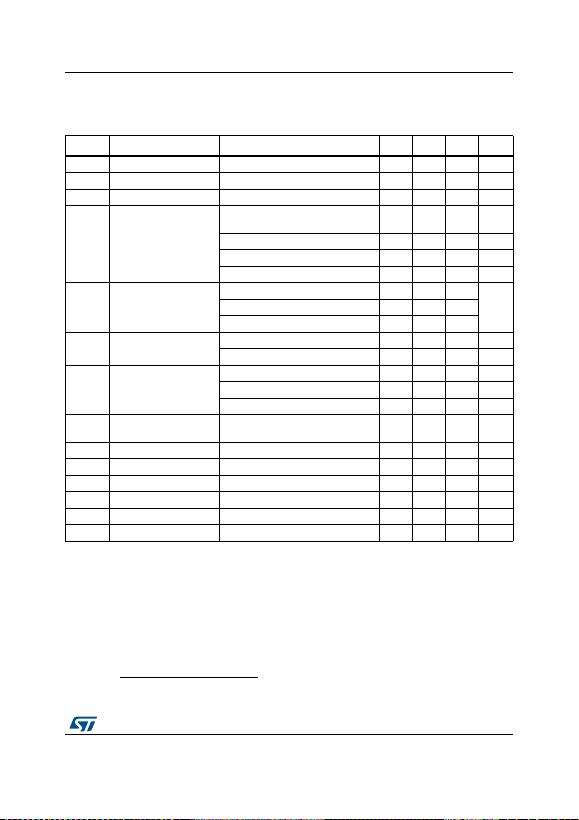DocID2143 Rev 33 9/59
L78 Electrical ch ara ct er istics
59
V
I
= 14 V, I
O
= 1 A, T
J
= 0 to 125 °C (L7808AC), T
J
= -40 to 125 °C (L7808AB), unl ess
otherwise specified
(c)
.
c. Minimum load current for regulation is 5 mA.
Table 5. Electrical characteristics of L7808A
Symbol Parameter Test conditions Min. Typ. Max. Unit
V
O
Output voltage T
J
= 25°C 7.84 8 8.16 V
V
O
Output voltage I
O
= 5 mA to 1 A, V
I
= 10.6 to 21 V 7.7 8 8.3 V
V
O
Output voltage I
O
= 1 A, V
I
= 21 to 23 V, T
J
= 25°C 7 .7 8 8.3 V
ΔV
O (1)
Line regulation
V
I
= 10.6 to 25 V, I
O
= 500 mA,
T
J
= 25°C 12 80 mV
V
I
= 11 to 17 V 15 80 mV
V
I
= 11 to 17 V, T
J
= 25°C 5 40 mV
V
I
= 10.4 to 23 V, T
J
= 25°C 12 80 mV
ΔV
O (1)
I
O
= 5 mA t o 1 A 25 100
mVI
O
= 5 mA to 1.5 A, T
J
= 25°C 30 100
I
O
= 250 to 750 mA 10 50
I
q
Quiescent current T
J
= 25°C 4.3 6 mA
6mA
ΔI
q
Quiescent current change
V
I
= 11 to 23 V, I
O
= 500 mA 0.8 mA
V
I
= 10.6 to 23 V, T
J
= 25°C 0.8 mA
I
O
= 5 mA to 1 A 0.5 mA
SVR Supply voltage rejection V
I
= 11.5 to 21.5 V, f = 120 Hz,
I
O
= 500 mA 62 dB
V
d
Dropout voltage I
O
= 1 A, T
J
= 25°C 2 V
eN Output noise voltage T
A
= 25°C, B =10 Hz to 100 kHz 10 µV/V
O
R
O
Output resistance f = 1 kHz 18 mΩ
I
sc
Short circuit current V
I
= 35 V, T
A
= 25°C 0.2 A
I
scp
Short circuit peak current T
J
= 25°C 2.2 A
ΔV
O
/ΔT Output voltage drift -0.8 mV/°C
1. Load and line regulation are specified at const ant junction temperature. Changes in V
O
due to heating effects must be
taken into account separately. Pulse testing with low duty cycle is used.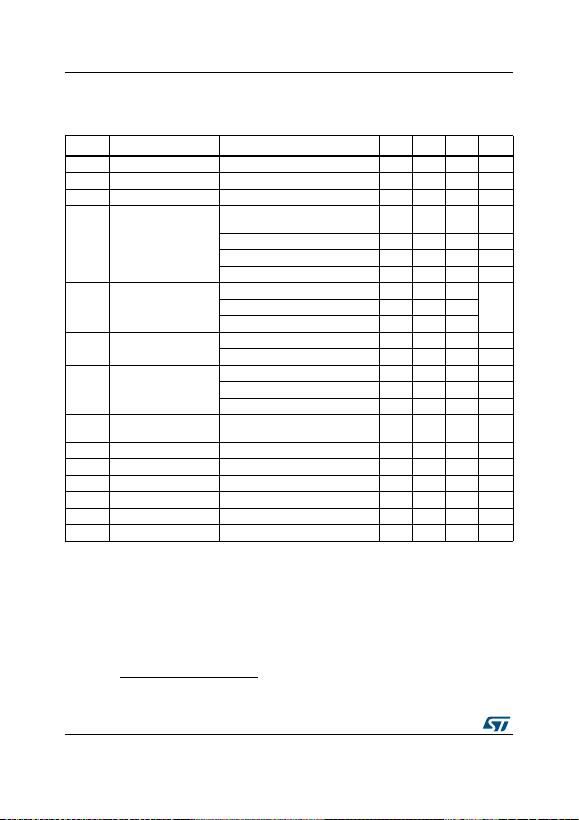Ele ctrical characteristics L78
10/59 DocID2143 Rev 33
V
I
= 15 V, I
O
= 1 A, T
J
= 0 to 125 °C (L7809AC), T
J
= -40 to 125 °C (L7809AB), unl ess
otherwise specified
(d)
.
d. Minimum load current for regulation is 5 mA.
Table 6. Electrical characteristics of L7809A
Symbol Parameter Test conditions Min. Typ. Max. Unit
V
O
Output voltage T
J
= 25°C 8.82 9 9.18 V
V
O
Output voltage I
O
= 5 mA to 1 A, V
I
= 10.6 to 22 V 8.65 9 9.35 V
V
O
Output voltage I
O
= 1 A, V
I
= 22 to 24 V, T
J
= 25°C 8.65 9 9.35 V
ΔV
O (1)
Line regulation
V
I
= 10.6 to 25 V, I
O
= 500 mA,
T
J
= 25°C 12 90 mV
V
I
= 11 to 17 V 15 90 mV
V
I
= 11 to 17 V, T
J
= 25°C 5 45 mV
V
I
= 11.4 to 23 V, T
J
= 25°C 12 90 mV
ΔV
O (1)
I
O
= 5 mA t o 1 A 25 100
mVI
O
= 5 mA to 1.5 A, T
J
= 25°C 30 100
I
O
= 250 to 750 mA 10 50
I
q
Quiescent current T
J
= 25°C 4.3 6 mA
6mA
ΔI
q
Quiescent current change
V
I
= 11 to 25 V, I
O
= 500 mA 0.8 mA
V
I
= 10.6 to 23 V, T
J
= 25°C 0.8 mA
I
O
= 5 mA to 1 A 0.5 mA
SVR Supply voltage rejection V
I
= 11.5 to 21.5 V, f = 120 Hz,
I
O
= 500 mA 61 dB
V
d
Dropout voltage I
O
= 1 A, T
J
= 25°C 2 V
eN Output noise voltage T
A
= 25°C, B =10 Hz to 100 kHz 10 µV/V
O
R
O
Output resistance f = 1 kHz 18 mΩ
I
sc
Short circuit current V
I
= 35 V, T
A
= 25°C 0.2 A
I
scp
Short circuit peak current T
J
= 25°C 2.2 A
ΔV
O
/ΔT Output voltage drift -0.8 mV/°C
1. Load and line regulation are specified at const ant junction temperature. Changes in V
O
due to heating effects must be
taken into account separately. Pulse testing with low duty cycle is used.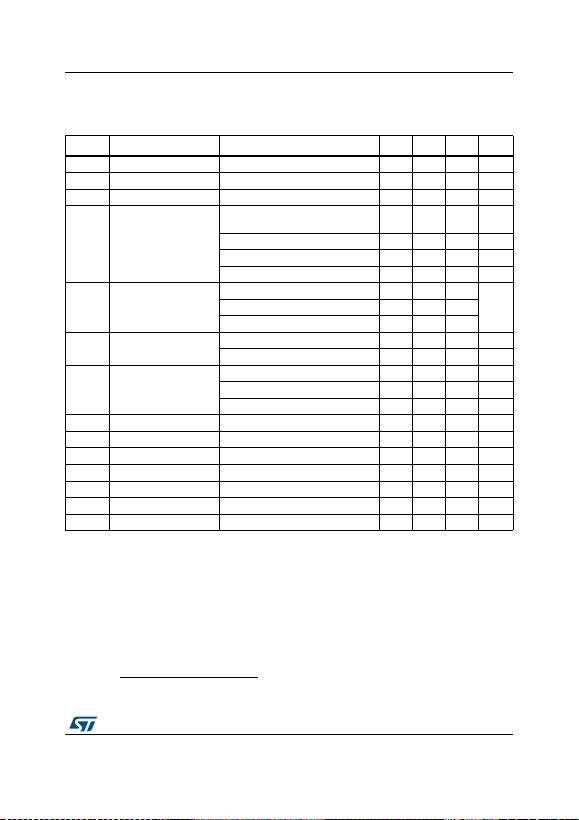DocID2143 Rev 33 11/59
L78 Electrical ch ara ct er istics
59
V
I
= 19 V, I
O
= 1 A, T
J
= 0 to 125 °C (L7812AC), T
J
= -40 to 125 °C (L7812AB), unl ess
otherwise specified
(e)
.
e. Minimum load current for regulation is 5 mA.
Table 7. Electrical characteristics of L7812A
Symbol Parameter Test conditions Min. Typ. Max. Unit
V
O
Output voltage T
J
= 25°C 11.75 12 12.25 V
V
O
Output voltage I
O
= 5 mA to 1 A, V
I
= 14.8 to 25 V 11.5 12 12.5 V
V
O
Output voltage I
O
= 1 A, V
I
= 25 to 27 V, T
J
= 25°C 11.5 12 12.5 V
ΔV
O (1)
Line regulation
V
I
= 14.8 to 30 V, I
O
= 500 mA,
T
J
= 25°C 13 120 mV
V
I
= 16 to 12 V 16 120 mV
V
I
= 16 to 12 V, T
J
= 25°C 6 60 mV
V
I
= 14.5 to 27 V, T
J
= 25°C 13 120 mV
ΔV
O (1)
I
O
= 5 mA t o 1 A 25 100
mVI
O
= 5 mA to 1.5 A, T
J
= 25°C 30 100
I
O
= 250 to 750 mA 10 50
I
q
Quiescent current T
J
= 25°C 4.4 6 mA
6mA
ΔI
q
Quiescent current change
V
I
= 15 to 30 V, I
O
= 500 mA 0.8 mA
V
I
= 14.8 to 27 V, T
J
= 25°C 0.8 mA
I
O
= 5 mA to 1 A 0.5 mA
SVR Supply voltage rejection V
I
= 15 to 25 V, f = 120 Hz, I
O
= 500 mA 60 dB
V
d
Dropout voltage I
O
= 1 A, T
J
= 25°C 2 V
eN Output noise voltage T
A
= 25°C, B = 10 Hz to 100 kHz 10 µV/V
O
R
O
Output resistance f = 1 kHz 18 mΩ
I
sc
Short circuit current V
I
= 35 V, T
A
= 25°C 0.2 A
I
scp
Short circuit peak current T
J
= 25°C 2.2 A
ΔV
O
/ΔT Output voltage drift -1 m V/°C
1. Load and line regulation are specified at const ant junction temperature. Changes in V
O
due to heating effects must be
taken into account separately. Pulse testing with low duty cycle is used.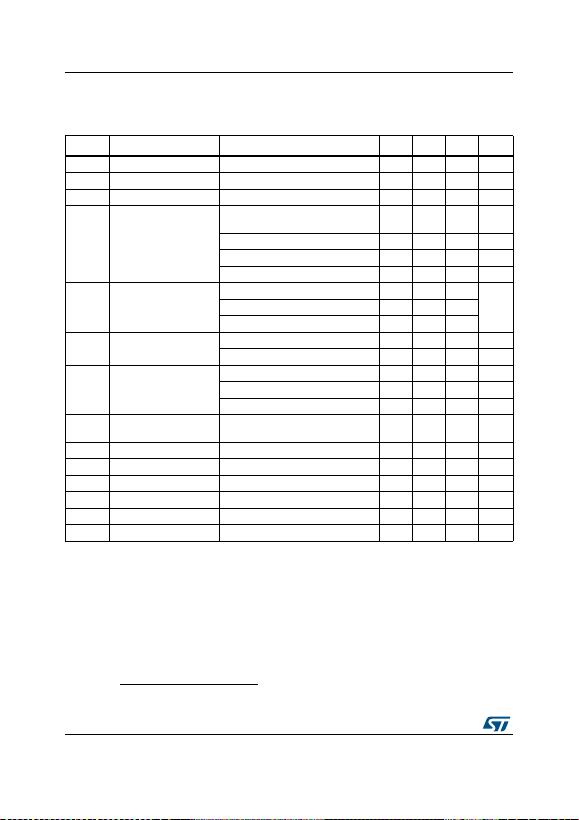Ele ctrical characteristics L78
12/59 DocID2143 Rev 33
V
I
= 23 V, I
O
= 1 A, T
J
= 0 to 125 °C (L7815AC), T
J
= -40 to 125 °C (L7815AB), unl ess
otherwise specified
(f)
.
f. Minimum load current for regulation is 5 mA.
Table 8. Electrical characteristics of L7815A
Symbol Parameter Test conditions Min. Typ. Max. Unit
V
O
Output voltage T
J
= 25°C 14.7 15 15.3 V
V
O
Output voltage I
O
= 5 mA to 1 A, V
I
= 17.9 to 28 V 14.4 15 15.6 V
V
O
Output voltage I
O
= 1 A, V
I
= 28 to 30 V, T
J
= 25°C 14.4 15 15.6 V
ΔV
O (1)
Line regulation
V
I
= 17.9 to 30 V, I
O
= 500 mA,
T
J
= 25°C 13 150 mV
V
I
= 20 to 26 V 16 150 mV
V
I
= 20 to 26 V, T
J
= 25°C 6 75 mV
V
I
= 17.5 to 30 V, T
J
= 25°C 13 150 mV
ΔV
O (1)
I
O
= 5 mA t o 1 A 25 100
mVI
O
= 5 mA to 1.5 A, T
J
= 25°C 30 100
I
O
= 250 to 750 mA 10 50
I
q
Quiescent current T
J
= 25°C 4.4 6 mA
6mA
ΔI
q
Quiescent current change
V
I
= 17.5 to 30 V, I
O
= 500 mA 0.8 mA
V
I
= 17.5 to 30 V, T
J
= 25°C 0.8 mA
I
O
= 5 mA to 1 A 0.5 mA
SVR Supply voltage rejection V
I
= 18.5 to 28.5 V, f = 120 Hz,
I
O
= 500 mA 58 dB
V
d
Dropout voltage I
O
= 1 A, T
J
= 25°C 2 V
eN Output noise voltage T
A
= 25°C, B = 10Hz to 100 kHz 10 µV/V
O
R
O
Output resistance f = 1 kHz 19 mΩ
I
sc
Short circuit current V
I
= 35 V, T
A
= 25°C 0.2 A
I
scp
Short circuit peak current T
J
= 25°C 2.2 A
ΔV
O
/ΔT Output voltage drift -1 m V/°C
1. Load and line regulation are specified at const ant junction temperature. Changes in V
O
due to heating effects must be
taken into account separately. Pulse testing with low duty cycle is used.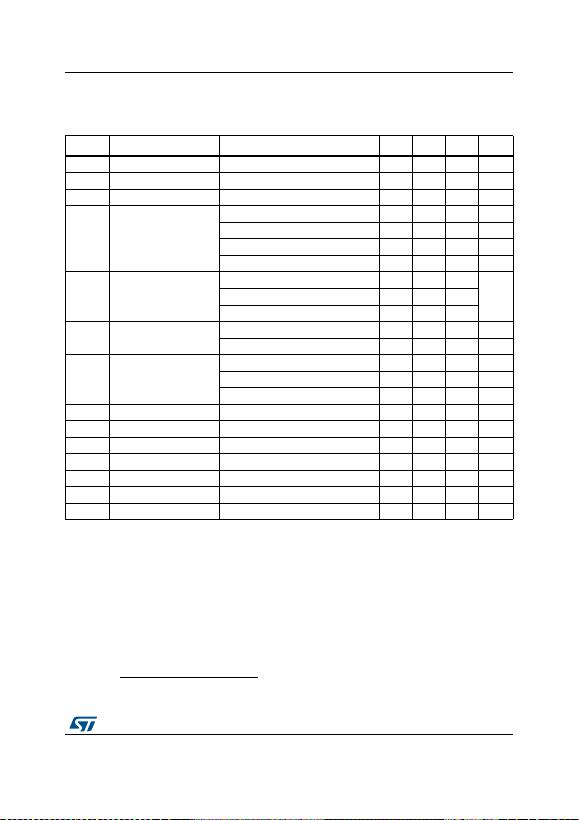DocID2143 Rev 33 13/59
L78 Electrical ch ara ct er istics
59
V
I
= 33 V, I
O
= 1 A, T
J
= 0 to 125 °C (L7824AC), T
J
= -40 to 125 °C (L7824AB), unl ess
otherwise specified
(g)
.
g. Minimum load current for regulation is 5 mA.
Table 9. Electrical characteristics of L7824A
Symbol Parameter Test conditions Min. Typ. Max. Unit
V
O
Output voltage T
J
= 25°C 23.5 24 24.5 V
V
O
Output voltage I
O
= 5 mA to 1 A, V
I
= 27.3 to 37 V 23 24 25 V
V
O
Output voltage I
O
= 1 A, V
I
= 37 to 38 V, T
J
= 25°C 23 24 25 V
ΔV
O (1)
Line regulation
V
I
= 27 to 38 V, I
O
= 500 mA, T
J
= 25°C 31 240 mV
V
I
= 30 to 36 V 35 200 mV
V
I
= 30 to 36 V, T
J
= 25°C 14 120 mV
V
I
= 26.7 to 38 V, T
J
= 25°C 31 240 mV
ΔV
O (1)
I
O
= 5 mA t o 1 A 25 100
mVI
O
= 5 mA to 1.5 A, T
J
= 25°C 30 100
I
O
= 250 to 750 mA 10 50
I
q
Quiescent current T
J
= 25°C 4.6 6 mA
6mA
ΔI
q
Quiescent current change
V
I
= 27.3 to 38 V, I
O
= 500 mA 0.8 mA
V
I
= 27.3 to 38 V, T
J
= 25°C 0.8 mA
I
O
= 5 mA to 1 A 0.5 mA
SVR Supply voltage rejection V
I
= 28 to 38 V, f = 120 Hz, I
O
= 500 mA 54 dB
V
d
Dropout voltage I
O
= 1 A, T
J
= 25°C 2 V
eN Output noise voltage T
A
= 25°C, B = 10 Hz to 100 kHz 10 µV/V
O
R
O
Output resistance f = 1 kHz 20 mΩ
I
sc
Short circuit current V
I
= 35 V, T
A
= 25°C 0.2 A
I
scp
Short circuit peak current T
J
= 25°C 2.2 A
ΔV
O
/ΔT Output voltage drift -1.5 mV/°C
1. Load and line regulation are specified at const ant junction temperature. Changes in V
O
due to heating effects must be
taken into account separately. Pulse testing with low duty cycle is used.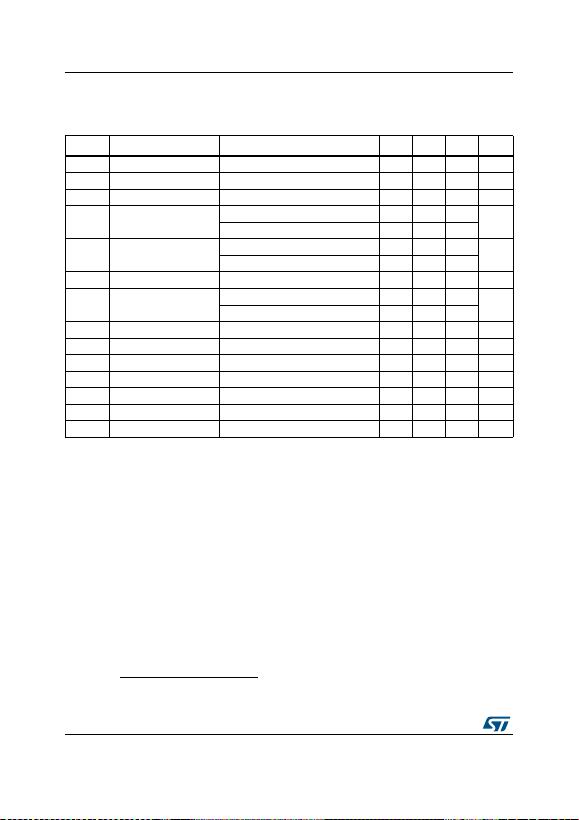Ele ctrical characteristics L78
14/59 DocID2143 Rev 33
Refer to the test circuits, T
J
= 0 to 125 °C, V
I
= 10 V, I
O
= 500 mA, C
I
= 0. 33 µF, C
O
= 0.1 µF
unless otherwise specified
(h)
.
h. Minimum load current for regulation is 5 mA.
Table 10. Electrical characteristics of L7805C
Symbol Parameter Test conditions Min. Typ. Max. Unit
V
O
Output voltage T
J
= 25°C 4.8 5 5.2 V
V
O
Output voltage I
O
= 5 mA to 1 A, V
I
= 7 to 18 V 4.75 5 5.25 V
V
O
Output voltage I
O
= 1 A, V
I
= 18 to 20V, T
J
= 25°C 4.75 5 5.25 V
ΔV
O(1)
Line regulation V
I
= 7 to 25 V, T
J
= 25°C 3 100 mV
V
I
= 8 to 12 V, T
J
= 25°C 1 50
ΔV
O(1)
O
= 5 mA to 1.5 A, T
J
= 25°C 100 mV
I
O
= 250 to 750 mA, T
J
= 25°C 50
I
d
Quiescent current T
J
= 25°C 8 mA
ΔI
d
Quiescent current change I
O
= 5 mA to 1 A 0.5 mA
V
I
= 7 to 23 V 0.8
ΔV
O
/ΔT Output voltage drift I
O
= 5 mA -1.1 mV/°C
eN Output noise voltage B = 10 Hz to 100 kHz, T
J
= 25°C 40 µV/V
O
SVR Supply voltage rejection V
I
= 8 to 18 V, f = 120 Hz 62 dB
V
d
Dropout voltage I
O
= 1 A, T
J
= 25°C 2 V
R
O
Output resistance f = 1 kHz 17 mΩ
I
sc
Short circuit current V
I
= 35 V, T
J
= 25°C 0.75 A
I
scp
Short circuit peak current T
J
= 25°C 2.2 A
1. Load and line regulation are specified at const ant junction temperature. Changes in V
O
due to heating effects must be
taken into account separately. Pulse testing with low duty cycle is used.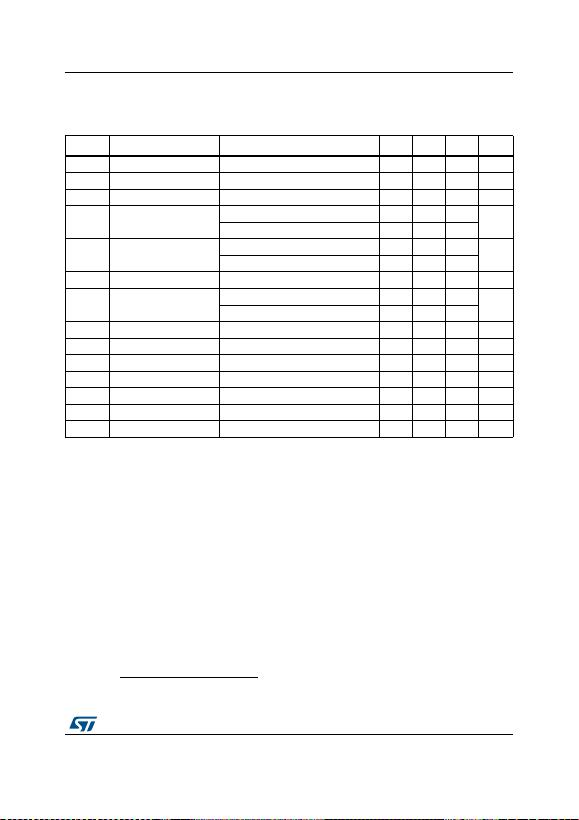DocID2143 Rev 33 15/59
L78 Electrical ch ara ct er istics
59
Refer to the test circuits, T
J
= 0 to 125 °C, V
I
= 11 V, I
O
= 500 mA, C
I
= 0. 3 3 µF, C
O
= 0.1 µF
unless otherwise specified
(i)
.
i. Minimum load current for regulation is 5 mA.
Table 11. Electrical characteristics of L7806C
Symbol Parameter Test conditions Min. Typ. Max. Unit
V
O
Output voltage T
J
= 25°C 5.75 6 6.25 V
V
O
Output voltage I
O
= 5 mA to 1 A, V
I
= 8 to 19 V 5.7 6 6.3 V
V
O
Output voltage I
O
= 1 A, V
I
= 19 to 21 V, T
J
= 25°C 5.7 6 6.3 V
ΔV
O(1)
Line regulation V
I
= 8 to 25 V, T
J
= 25°C 120 mV
V
I
= 9 to 13 V, T
J
= 25°C 60
ΔV
O(1)
O
= 5 mA to 1.5 A, T
J
= 25°C 120 mV
I
O
= 250 to 750 mA, T
J
= 25°C 60
I
d
Quiescent current T
J
= 25°C 8 mA
ΔI
d
Quiescent current change I
O
= 5 mA to 1 A 0.5 mA
V
I
= 8 to 24 V 1.3
ΔV
O
/ΔT Output voltage drift I
O
= 5 mA -0.8 mV/°C
eN Output noise voltage B = 10 Hz to 100 kHz, T
J
= 25°C 45 µV/V
O
SVR Supply voltage rejection V
I
= 9 to 19 V, f = 120 Hz 59 dB
V
d
Dropout voltage I
O
= 1 A, T
J
= 25°C 2 V
R
O
Output resistance f = 1 kHz 19 mΩ
I
sc
Short circuit current V
I
= 35 V, T
J
= 25°C 0.55 A
I
scp
Short circuit peak current T
J
= 25°C 2.2 A
1. Load and line regulation are specified at const ant junction temperature. Changes in V
O
due to heating effects must be
taken into account separately. Pulse testing with low duty cycle is used.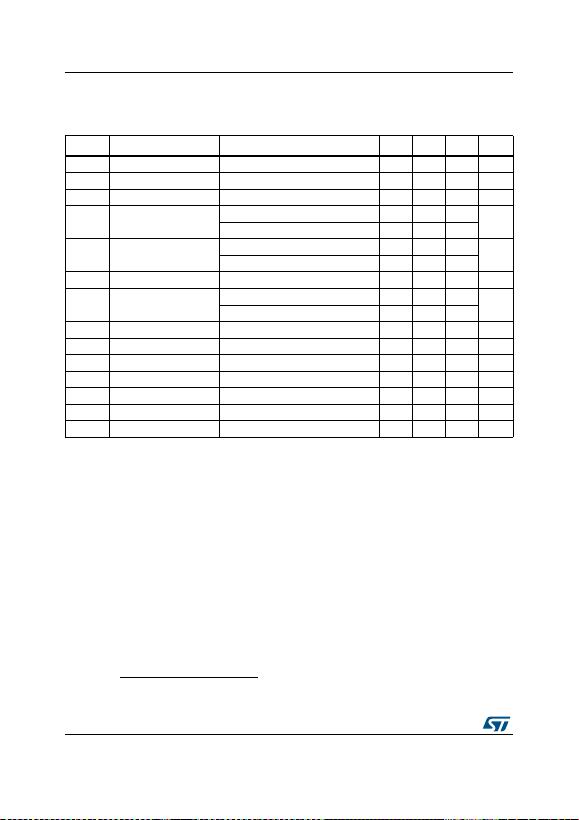Ele ctrical characteristics L78
16/59 DocID2143 Rev 33
Refer to the test circuits, T
J
= 0 to 125 °C, V
I
= 14 V, I
O
= 500 mA, C
I
= 0. 33 µF, C
O
= 0.1 µF
unless otherwise specified
(j)
.
j. Minimum load current for regulation is 5 mA.
Table 12. Electrical characteristics of L7808C
Symbol Parameter Test conditions Min. Typ. Max. Unit
V
O
Output voltage T
J
= 25°C 7.7 8 8.3 V
V
O
Output voltage I
O
= 5 mA to 1 A, V
I
= 10.5 to 21 V 7.6 8 8.4 V
V
O
Output voltage I
O
= 1 A, V
I
= 21 to 25 V, T
J
= 25°C 7.6 8 8.4 V
ΔV
O(1)
Line regulation V
I
= 10.5 to 25 V, T
J
= 25°C 160 mV
V
I
= 11 to 17 V, T
J
= 25°C 80
ΔV
O(1)
O
= 5 mA to 1.5 A, T
J
= 25°C 160 mV
I
O
= 250 to 750 mA, T
J
= 25°C 80
I
d
Quiescent current T
J
= 25°C 8 mA
ΔI
d
Quiescent current change I
O
= 5 mA to 1 A 0.5 mA
V
I
= 10.5 to 25 V 1
ΔV
O
/ΔT Output voltage drift I
O
= 5 mA -0.8 mV/°C
eN Output noise voltage B = 10 Hz to 100 kHz, T
J
= 25°C 52 µV/V
O
SVR Supply voltage rejection V
I
= 11.5 to 21.5 V, f = 120 Hz 56 dB
V
d
Dropout voltage I
O
= 1 A, T
J
= 25°C 2 V
R
O
Output resistance f = 1 kHz 16 mΩ
I
sc
Short circuit current V
I
= 35 V, T
J
= 25°C 0.45 A
I
scp
Short circuit peak current T
J
= 25°C 2.2 A
1. Load and line regulation are specified at const ant junction temperature. Changes in V
O
due to heating effects must be
taken into account separately. Pulse testing with low duty cycle is used.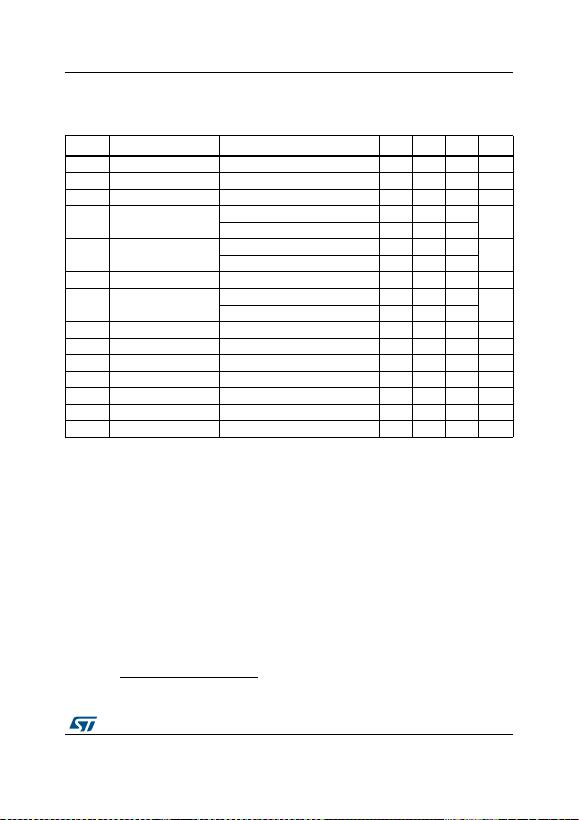DocID2143 Rev 33 17/59
L78 Electrical ch ara ct er istics
59
Refer to the test circuits, T
J
= 0 to 125 °C, V
I
= 14.5 V, I
O
= 500 mA, C
I
= 0.33 µF,
C
O
= 0.1 µF unless otherwise specified
(k)
.
k. Minimum load current for regulation is 5 mA.
Table 13. Electrical characteristics of L7885C
Symbol Parameter Test conditions Min. Typ. Max. Unit
V
O
Output voltage T
J
= 25°C 8.2 8.5 8.8 V
V
O
Output voltage I
O
= 5 mA to 1 A, V
I
= 11 to 21.5 V 8.1 8.5 8.9 V
V
O
Output voltage I
O
= 1 A, V
I
= 21.5 to 26 V, T
J
= 25°C 8.1 8.5 8.9 V
ΔV
O(1)
Line regulation V
I
= 11 to 27 V, T
J
= 25°C 160 mV
V
I
= 11.5 to 17.5 V, T
J
= 25°C 80
ΔV
O(1)
O
= 5 mA to 1.5 A, T
J
= 25°C 160 mV
I
O
= 250 to 750 mA, T
J
= 25°C 80
I
d
Quiescent current T
J
= 25°C 8 mA
ΔI
d
Quiescent current change I
O
= 5 mA to 1 A 0.5 mA
V
I
= 11 to 26 V 1
ΔV
O
/ΔT Output voltage drift I
O
= 5 mA -0.8 mV/°C
eN Output noise voltage B = 10 Hz to 100 kHz, T
J
= 25°C 55 µV/V
O
SVR Supply voltage rejection V
I
= 12 to 22 V, f = 120 Hz 56 dB
V
d
Dropout voltage I
O
= 1 A, T
J
= 25°C 2 V
R
O
Output resistance f = 1 kHz 16 mΩ
I
sc
Short circuit current V
I
= 35 V, T
J
= 25°C 0.45 A
I
scp
Short circuit peak current T
J
= 25°C 2.2 A
1. Load and line regulation are specified at const ant junction temperature. Changes in V
O
due to heating effects must be
taken into account separately. Pulse testing with low duty cycle is used.Ele ctrical characteristics L78
18/59 DocID2143 Rev 33
Refer to the test circuits, T
J
= 0 to 125 °C, V
I
= 15 V, I
O
= 500 mA, C
I
= 0. 33 µF, C
O
= 0.1 µF
unless otherwise specified
(l)
.
l. Minimum load current for regulation is 5 mA.
Table 14. Electrical characteristics of L7809C
Symbol Parameter Test conditions Min. Typ. Max. Unit
V
O
Output voltage T
J
= 25°C 8.64 9 9.36 V
V
O
Output voltage I
O
= 5 mA to 1 A, V
I
= 11.5 to 22 V 8.55 9 9.45 V
V
O
Output voltage I
O
= 1 A, V
I
= 22 to 26 V, T
J
= 25°C 8.55 9 9.45 V
ΔV
O(1)
Line regulation V
I
= 11.5 to 26 V, T
J
= 25°C 18 0 mV
V
I
= 12 to 18 V, T
J
= 25°C 90
ΔV
O(1)
O
= 5 mA to 1.5 A, T
J
= 25°C 180 mV
I
O
= 250 to 750 mA, T
J
= 25°C 90
I
d
Quiescent current T
J
= 25°C 8 mA
ΔI
d
Quiescent current change I
O
= 5 mA to 1 A 0.5 mA
V
I
= 11.5 to 26 V 1
ΔV
O
/ΔT Output voltage drift I
O
= 5 mA -1 mV/°C
eN Output noise voltage B = 10 Hz to 100 kHz, T
J
= 25°C 70 µV/V
O
SVR Supply voltage rejection V
I
= 12 to 23 V, f = 120 Hz 55 dB
V
d
Dropout voltage I
O
= 1 A, T
J
= 25°C 2 V
R
O
Output resistance f = 1 kHz 17 mΩ
I
sc
Short circuit current V
I
= 35 V, T
J
= 25°C 0.40 A
I
scp
Short circuit peak current T
J
= 25°C 2.2 A
1. Load and line regulation are specified at const ant junction temperature. Changes in V
O
due to heating effects must be
taken into account separately. Pulse testing with low duty cycle is used.